﻿ SuperShape 2D

# SuperShape 2D

 SuperShape 2D by Nicholas Breslow

• Where to find it:

• Add Procedural Layer / Procedural / Custom / Pattern /

•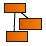NodeGraph / Right Mouse Click / Add Nodes / Procedural / Custom / Pattern /

SuperShape 2D is a powerful pattern generation algorithm based on the superformula. It is capable of creating a wide array through overlaying sinus and cosinus functions. Just a few (together with their settings) are shown below: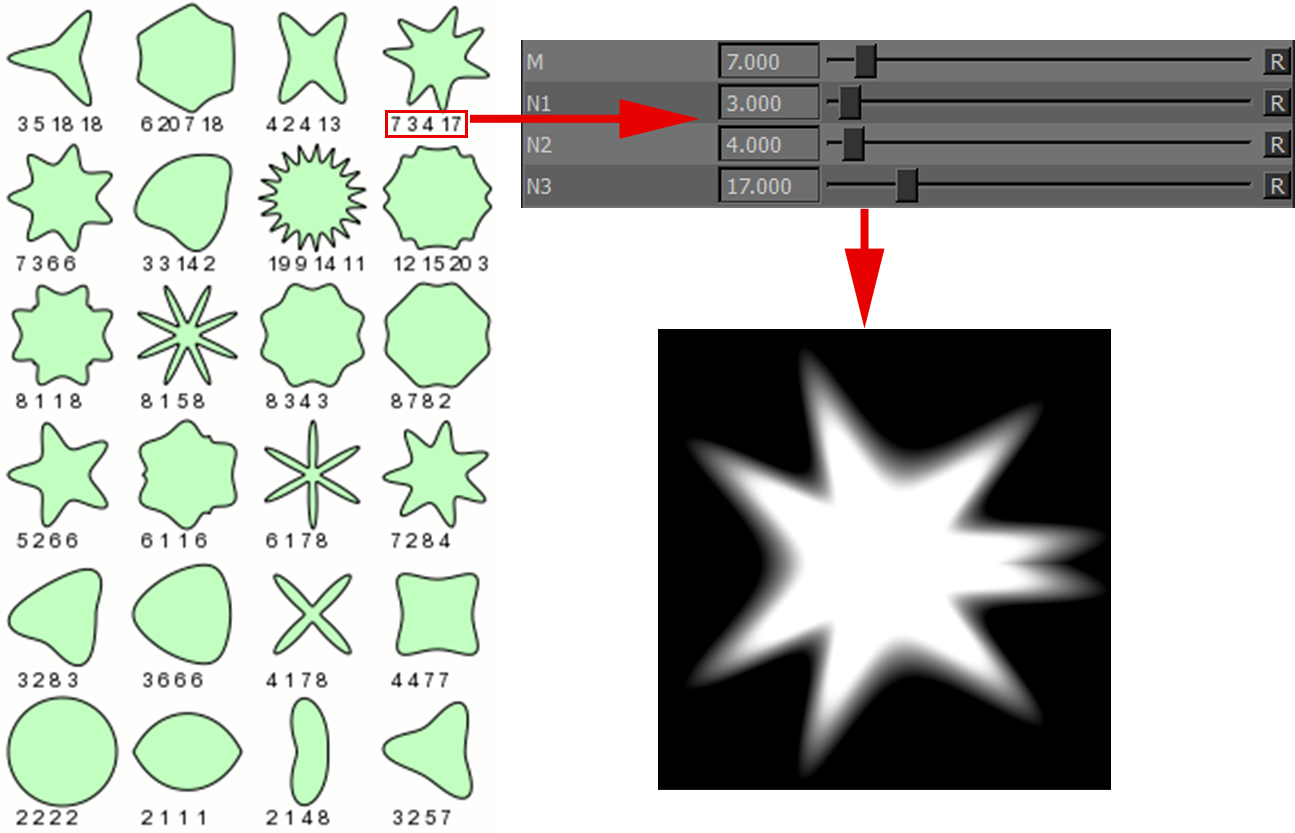Samples

Just a few of the many variations of the Supershape 2D Node: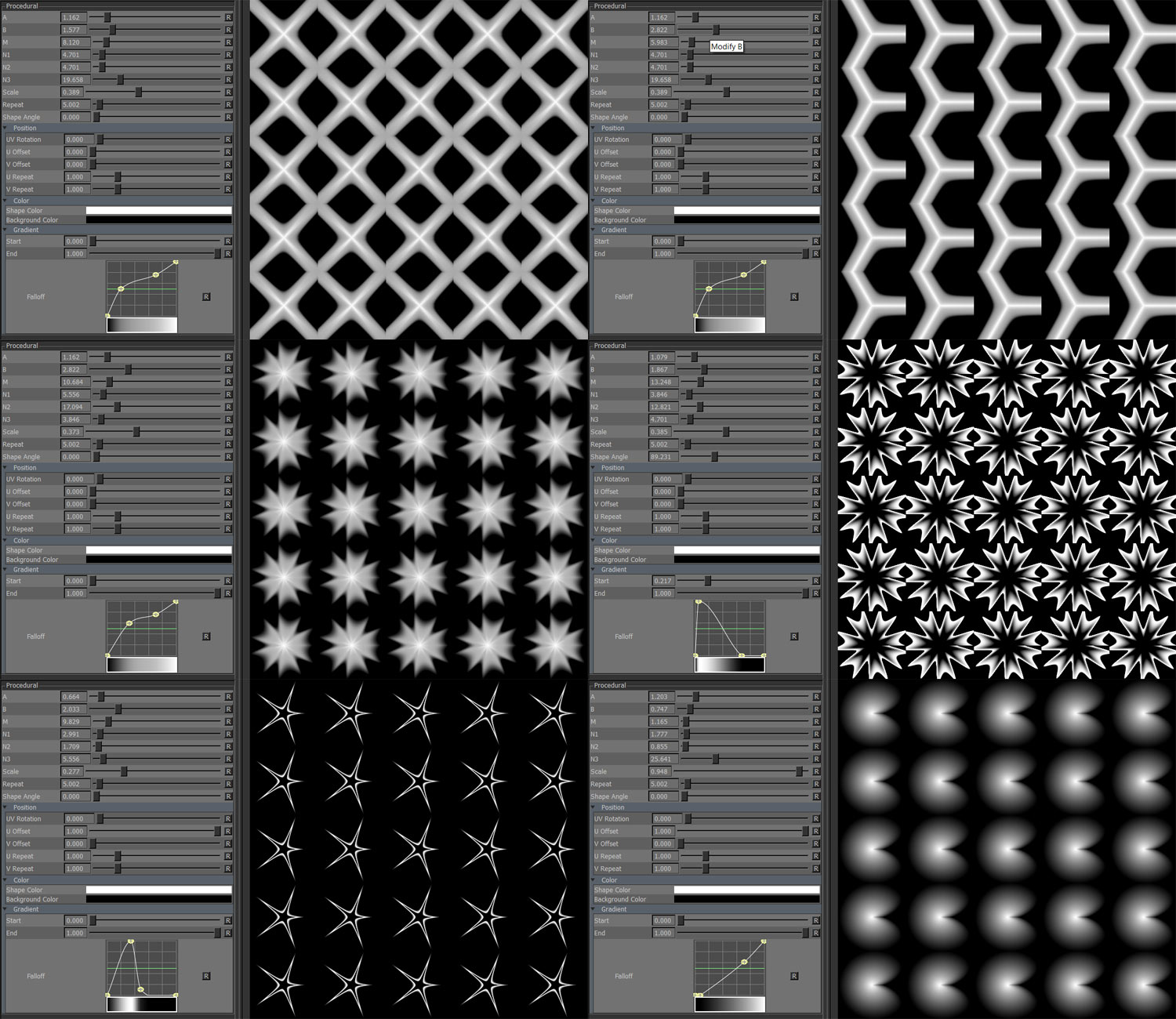Options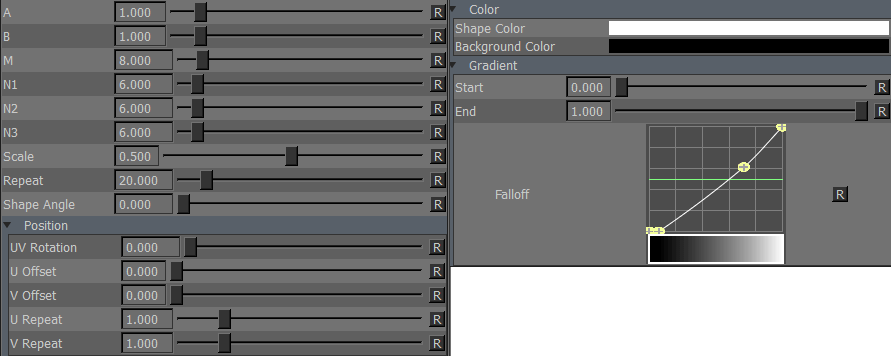Main

• A

A is a Divisor on the cosinus function of the superformula.

Shapes are generated by overlaying a cosinus and a sinus function.

Changing A therefore only scales parts of the generated shapes leading to asymmetry.

Increasing 'A' on a single Pulse (m=1)                                Increasing 'A' on multiple Pulses (m=8)

will scale the part generated by cosinus                             will scale the part generated by cosinus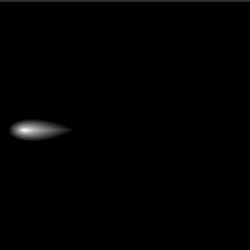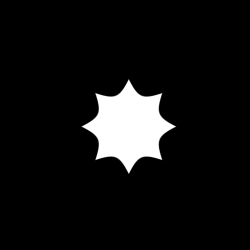• B

B is a Divisor on the sinus function of the superformula.

Shapes are generated by overlaying a cosinus and a sinus function.

Changing B therefore only scales parts of the generated shapes leading to asymmetry.

Increasing 'B' on a single Pulse (m=1)                                Increasing 'B' on multiple Pulses (m=8)

will scale the part generated by sinus                                 will scale the part generated by sinus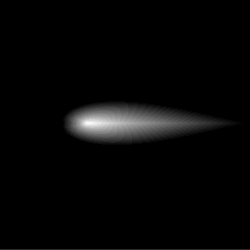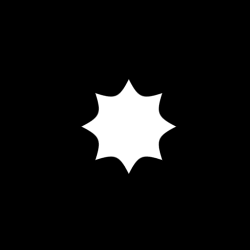• M

Increasing M adds rotational symmetry to the shape. Probably the most important setting of the node.

Full, even numbers result in symmetrical shapes. Asymmetric forms can be created by uneven numbers and using decimals.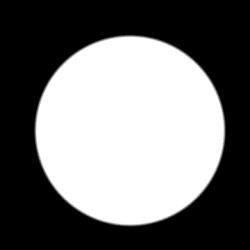• N1 / N2 / N3

If N1,N2 and N3 are equal but lower than 1.0, forms are increasingly pinched:

A pulse with equal N1,N2 and N3 values resulting in a pinched look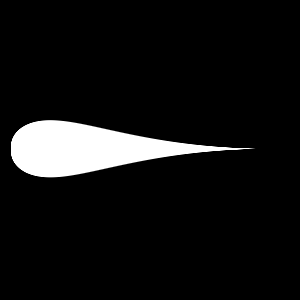If N1 is slightly larger than N2 and N3 then bloated forms result:

A pulse with equal N1 twice the size of N2 and N3 resulting in a bloated look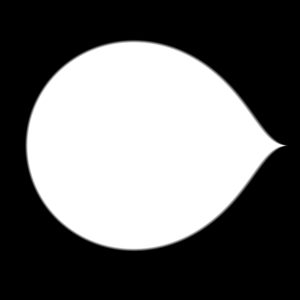Polygonal forms can be achieved with large values of N1 and large but equal numbers of N2 and N3.

For a Triangle shape use a N1 smaller than N2/N3, for quads use N1 with larger values than N2,N3.

Polygonal Shape with N1 = 95, N2+N3 = 60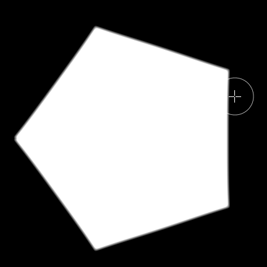Starfish Shapes can be created by using considerably smaller values of N1 than N2 + N3, but equal

N2 + N3 Values.

Starfish Shape with N1 = 20, N2+N3 = 50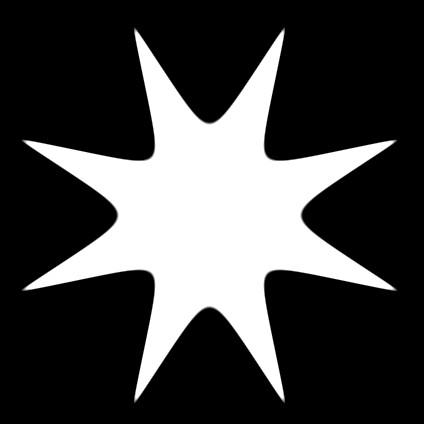• Scale

Scale of each shape. Decreasing shape size increases gap size between shapes.

• Repeat

The number of stripes to draw.

• Shape Angle

Rotating in degrees. Pivot is the center of each Shape (different from UV Rotation)

 Position

• UV Rotation

Rotating in degrees. Pivot is the center of each UV Tile / UDIM.

• U Offset

Translates shapes horizontally in UV Space.

• V Offset

Translates shapes vertically in UV Space.

• U Repeat

Multiplier against 'Repeat' for the number of shapes drawn horizontally in UV Space.

• V Repeat

Multiplier against 'Repeat' for the number of shapes drawn vertically in UV Space.

 Color

• Shape Color

Color of each shape.

• Background Color

Color of area around shape.

• Start

The Value range up to which Background Color is drawn.

• End

The Value representing the full Shape Color.

• Falloff

Remap Curve against the final result.

Created with the Personal Edition of HelpNDoc: Full-featured EBook editor